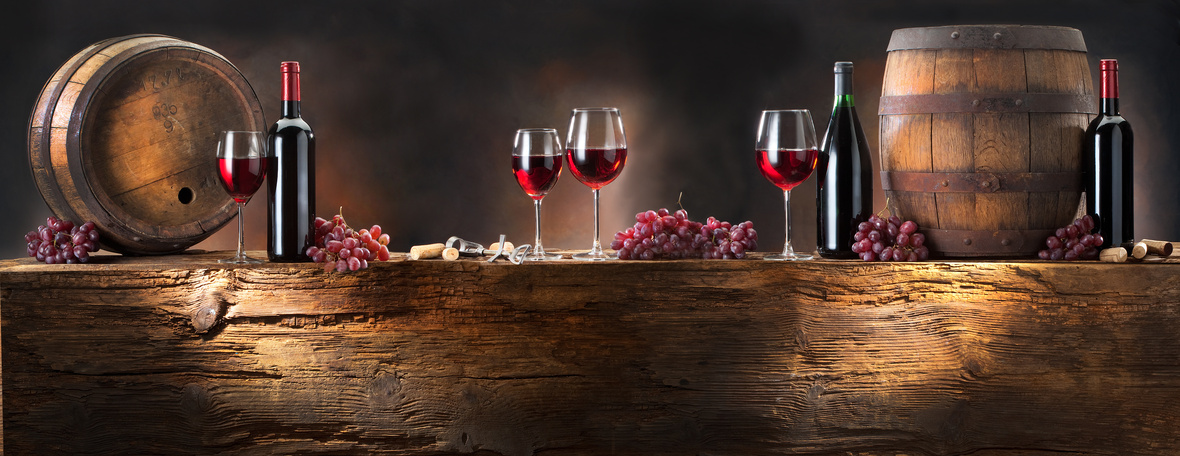# Wine Finds and Purposeful Virtual Connections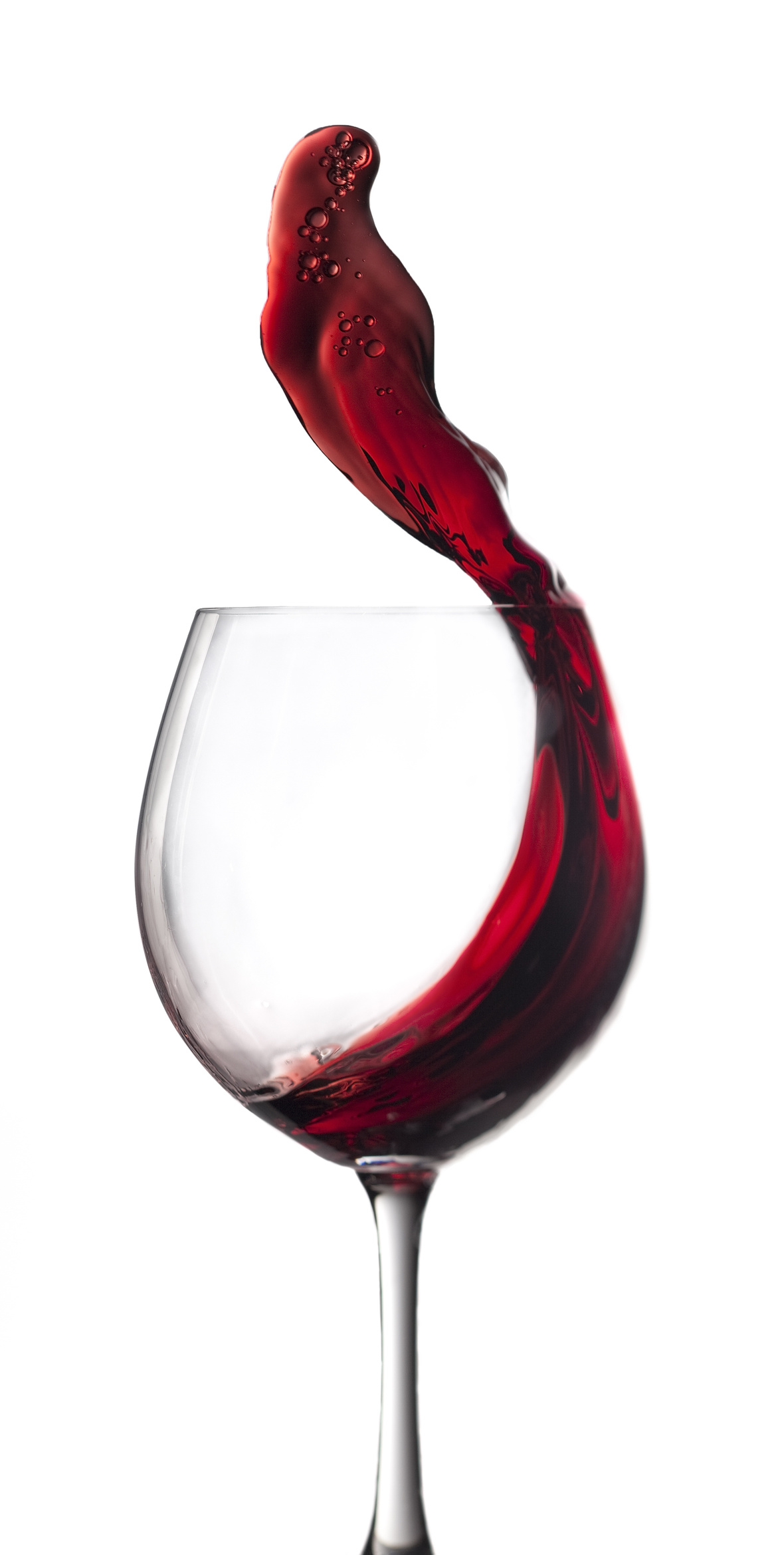Hi Wine Lover,

The Shall We Wine team is excited to share our monthly newsletter. Our goal is to introduce you to our favorite wines, winemakers, varietals, wine regions and places to buy and drink wine.

Cheers,

The Shall We Wine Team

 /* styles */

## HEIGHT OF SUMMERWe may not all be flocking to beaches, but we are occupying some serious internet space! Virtual connection healing, if you will.

Beneath the radiant sunshine of Summer 2020, the fundamental facets of quarantine unveils a mixed bag of embracing opportunities, dodging roadblocks and seeking comfort and security. But our tenacious spirit and durability is our muscle - made for quarantine.

Summertime amid a pandemic is weird and challenging to say the least, but glimmers of hope, fun and togetherness keeps us going! Can you believe we're more than halfway through the year?

So whether you're here to refresh your everyday beverage selections, update your routine or to simply see what else we recommend to "put you on", you best know it'll be a delectable treat to bring you even a bit of sunshine!

 table div table+table+table+table+table+table+table div table{width:100%;padding:0}table div table+table+table+table+table+table+table div table img{width:96.23%;padding:0;float:none}table div table+table+table+table+table+table+table div table td{width:100%;padding:0 1.88% 18px}/* styles */## PINUAGA VIRTUAL TASTING: THIS FRIDAY 7/24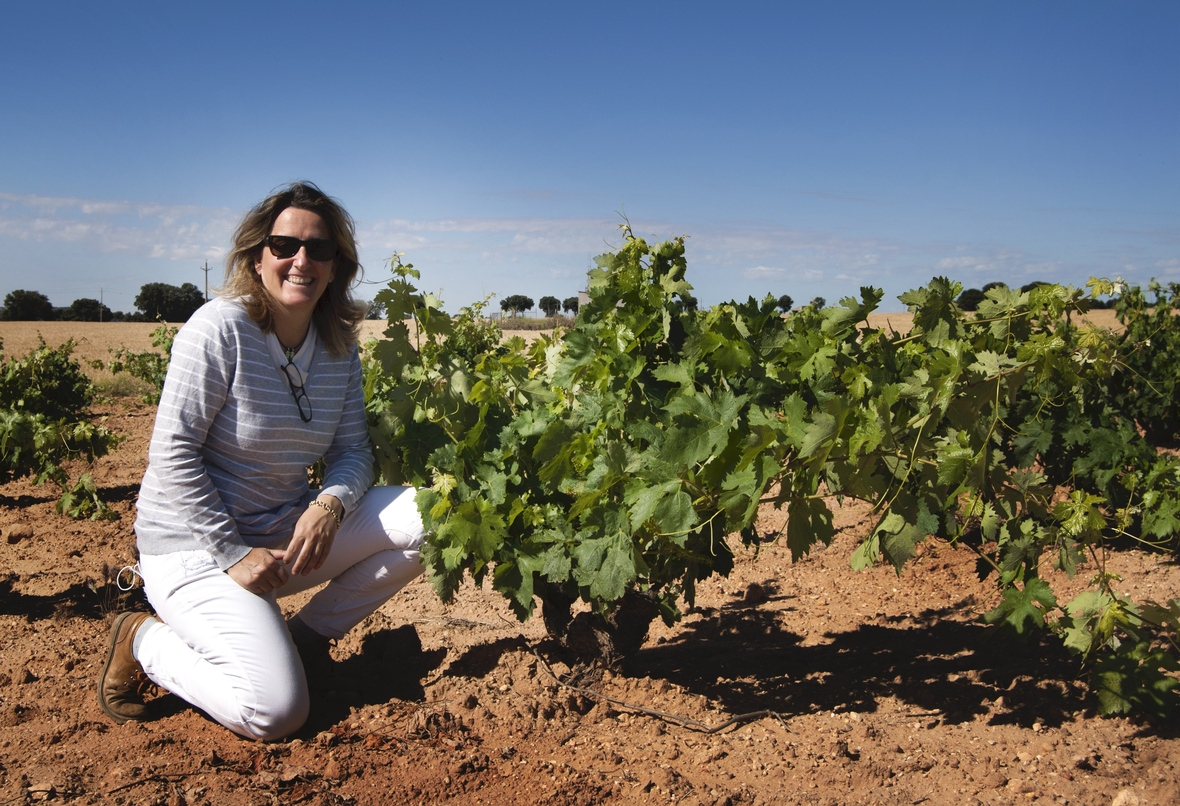Join Shall We Wine and Esther Pinauga of Pinuaga Winery in Toledo Spain for an exclusive virtual wine tasting and talk!

The Pinuaga Family has been making wines since 1960, with a focus on the Cencibel grape (an old, indigenous clone of Tempranillo) and always with a deep respect for the environment.

A remarkable woman, Esther not only manages the sales and business side of the winery business, she is also the President of the Spanish Organic Winegrowers Association.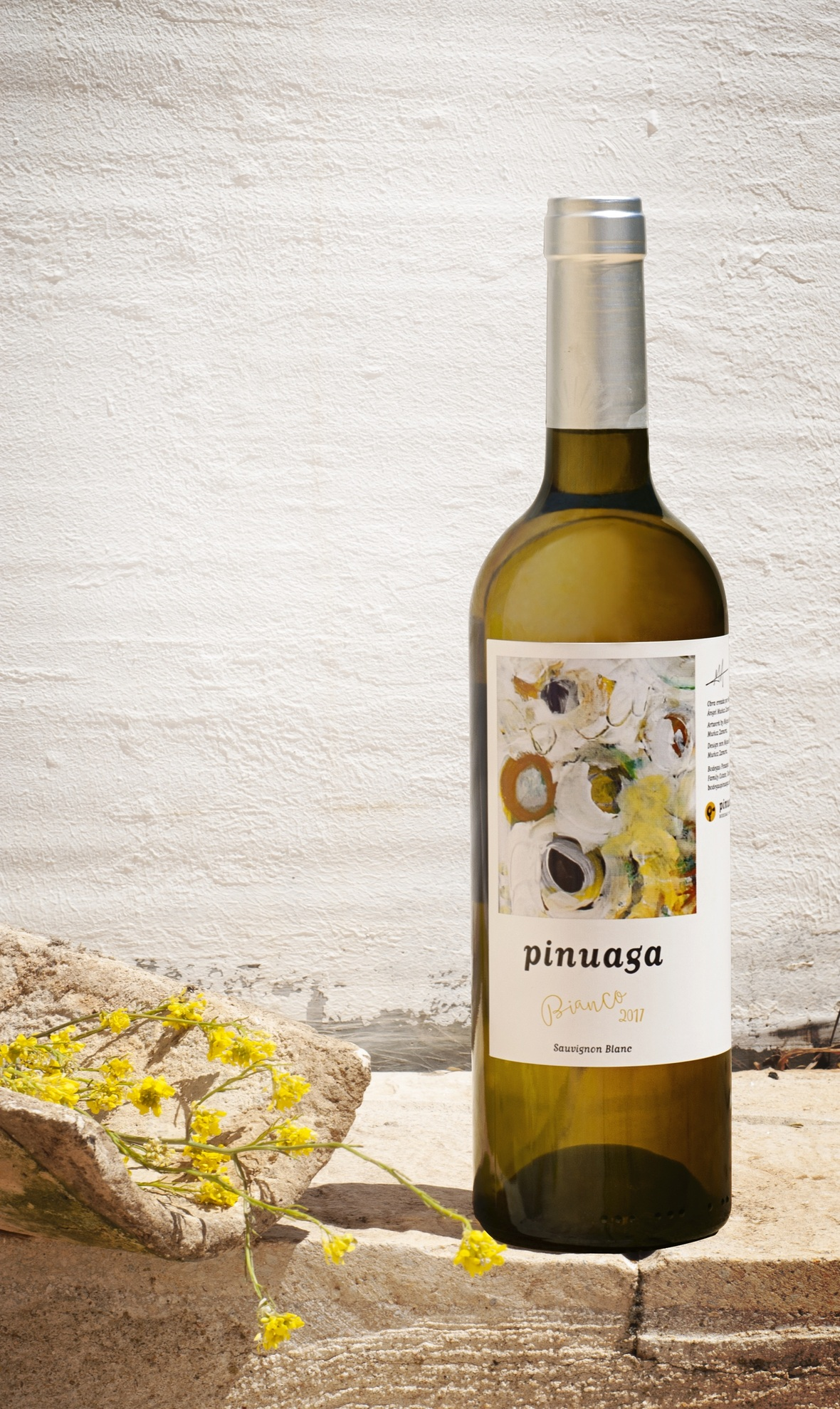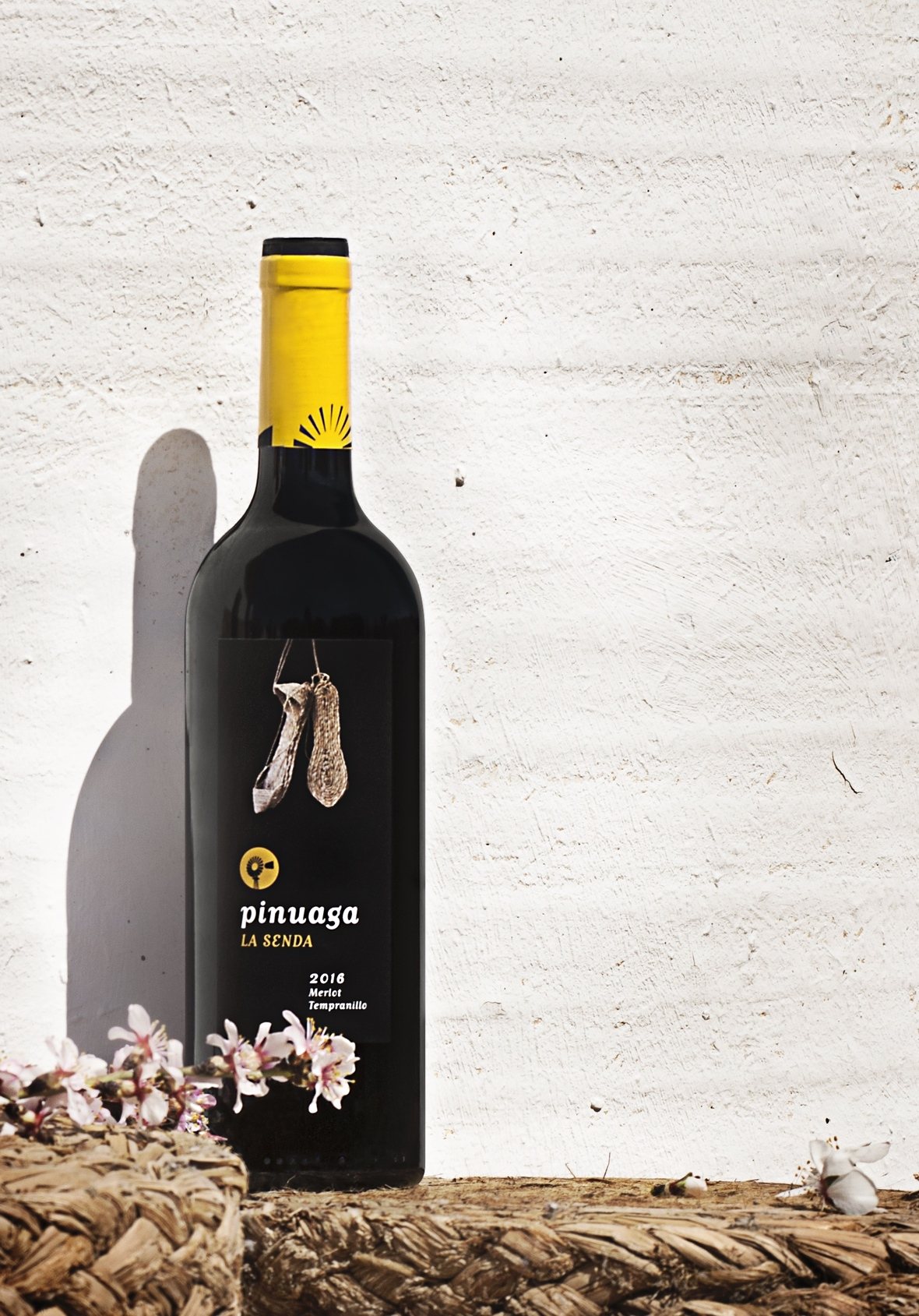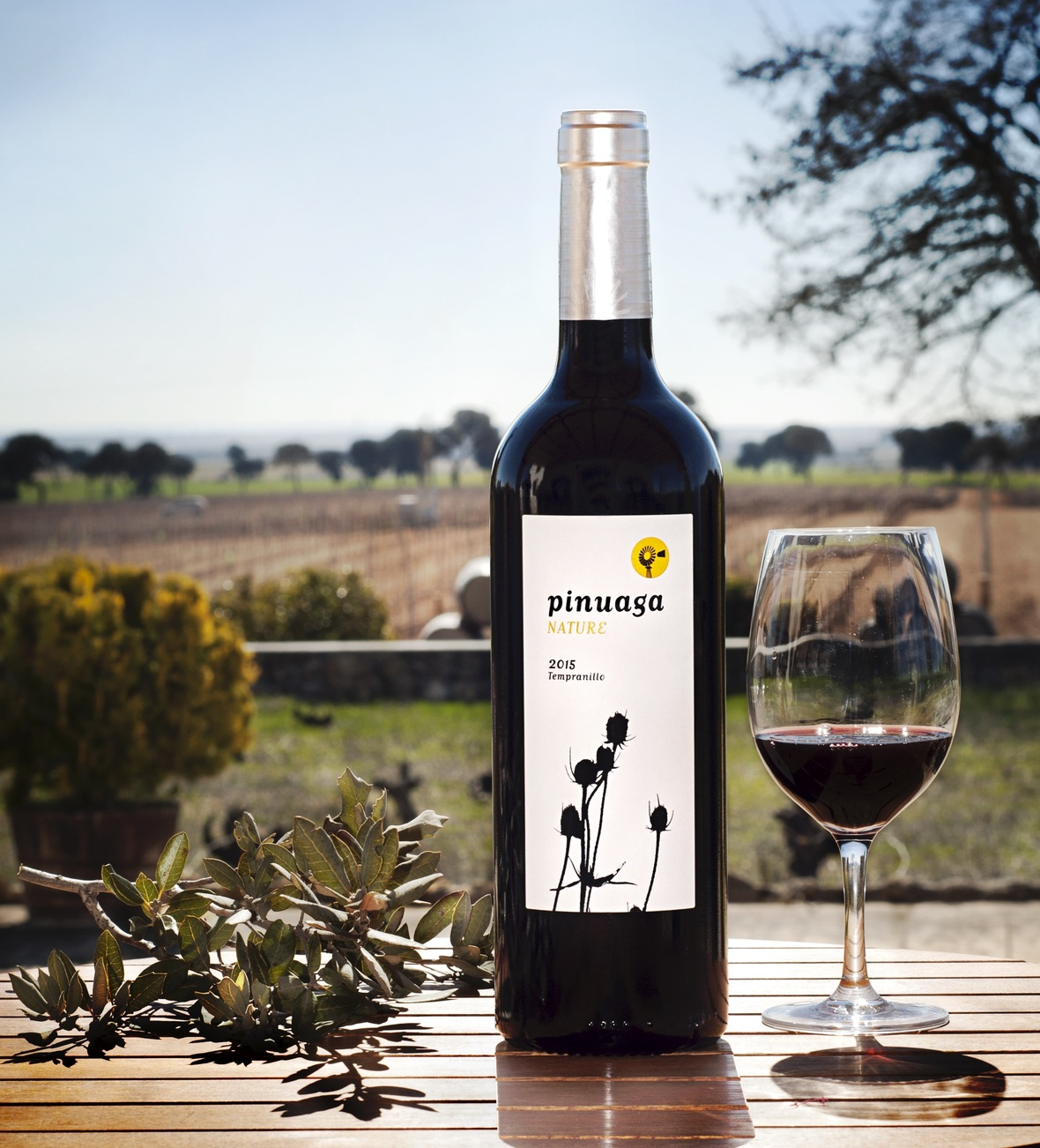table div table+table+table+table+table+table+table+table+table+table+table+table+table div table{width:100%;padding:0}table div table+table+table+table+table+table+table+table+table+table+table+table+table div table img{width:96.23%;padding:0;float:none}table div table+table+table+table+table+table+table+table+table+table+table+table+table div table td{width:100%;padding:0 1.88% 18px}/* styles */## SAFE SPACES: SWW AFTER PARTY CHATSThe intention of the "After Party" which follows our Cocktail Hours is to offer a safe space where we discuss current events, ask questions and surrender to courageous conversations.

The initial iterations of the "After Party" happened organically - a result of a community wanting to seek resources and comfort, but ultimately yearning to create and be part of a space of healing, support and nonjudgmental comradery.

With each "After Party" we have evolved, truly inspired by the community we are building.During one of our most recent "After Party" sessions in particular, we invited Alexandra Newman to share her work with the Violin Vigil for Elijah McClain.

On Saturday, July 11, 2020, string instrumentalists from across Chicago and the greater Chicagoland region gathered together in Oz Park for a peaceful musical assembly to commemorate the life and mourn the tragic death of Elijah McClain. As news of Elijah's murder became public recently, musicians playing the violin, viola, cello, bass, and other stringed instruments have gathered together in "Violin Vigils" in cities across the U.S. to mourn for Elijah and demand an end to police brutality and racism.Chicago Violin Vigil Honoring Elijah McClain: Somewhere Over the Rainbow * [ Click Here To Watch Video ]
 table div table+table+table+table+table+table+table+table+table+table+table+table+table+table+table+table+table+table div table{width:100%;padding:0}table div table+table+table+table+table+table+table+table+table+table+table+table+table+table+table+table+table+table div table img{width:96.23%;padding:0;float:none}table div table+table+table+table+table+table+table+table+table+table+table+table+table+table+table+table+table+table div table td{width:100%;padding:0 1.88% 18px}/* styles */## GUEST ON WINE & HIP HOP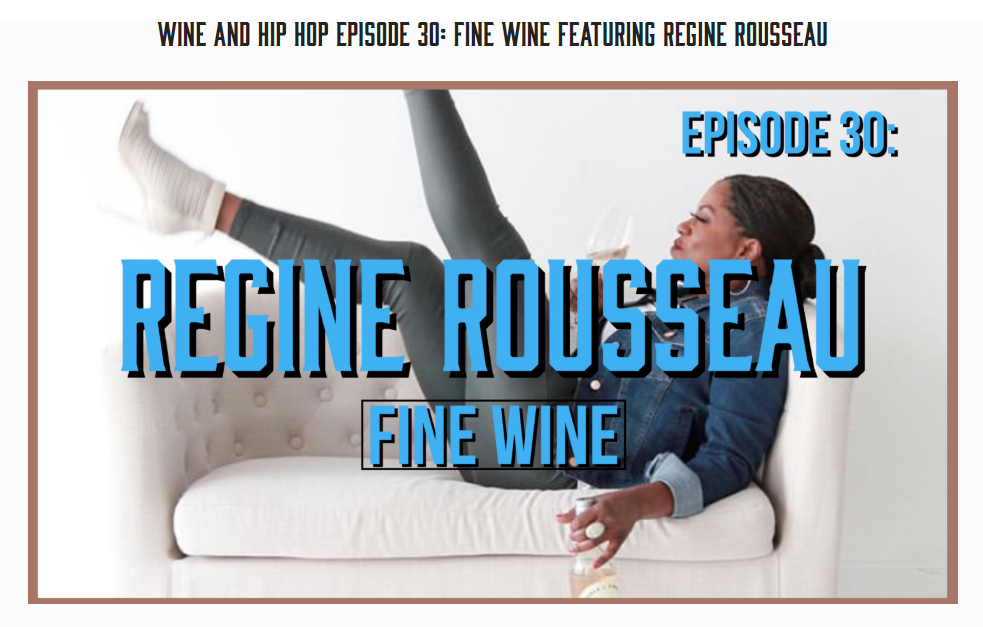This is easily in my Top 5 favorite Podcasts! Similar to what I do with poetry, using it to show common ground between elements of wine and the written word, Wine & Hip Hop does a superb job taking the subject matter of wine and putting it into the relatable context of Hip Hop music.

Note that this Podcast was recorded pre-Covid. Take a listen as I share my 2020 theme song and get schooled by Jermaine Stone about Hip Hop.

WARNING: This is grown folks talk while drinking grown folks wine!

 table div table+table+table+table+table+table+table+table+table+table+table+table+table+table+table+table+table+table+table+table+table div table{width:100%;padding:0}table div table+table+table+table+table+table+table+table+table+table+table+table+table+table+table+table+table+table+table+table+table div table img{width:96.23%;padding:0;float:none}table div table+table+table+table+table+table+table+table+table+table+table+table+table+table+table+table+table+table+table+table+table div table td{width:100%;padding:0 1.88% 18px}/* styles */## COCKTAILS FOR A CAUSEFemmebought is an amazing organization founded on one simple fact: support women in business.

What fun it was mixing signature mocktails and cocktails as a special guest for their Virtual Empowered Purse Gala!

Honored to have stood (virtually) alongside other movers and shakers who believe in the power of investing in women, particularly women of color. Being socially distant does not take away your ability to make a difference in your communities and challenge the status quo!

 table div table+table+table+table+table+table+table+table+table+table+table+table+table+table+table+table+table+table+table+table+table+table+table+table div table{width:100%;padding:0}table div table+table+table+table+table+table+table+table+table+table+table+table+table+table+table+table+table+table+table+table+table+table+table+table div table img{width:96.23%;padding:0;float:none}table div table+table+table+table+table+table+table+table+table+table+table+table+table+table+table+table+table+table+table+table+table+table+table+table div table td{width:100%;padding:0 1.88% 18px}/* styles */## IN CASE YOU MISSED IT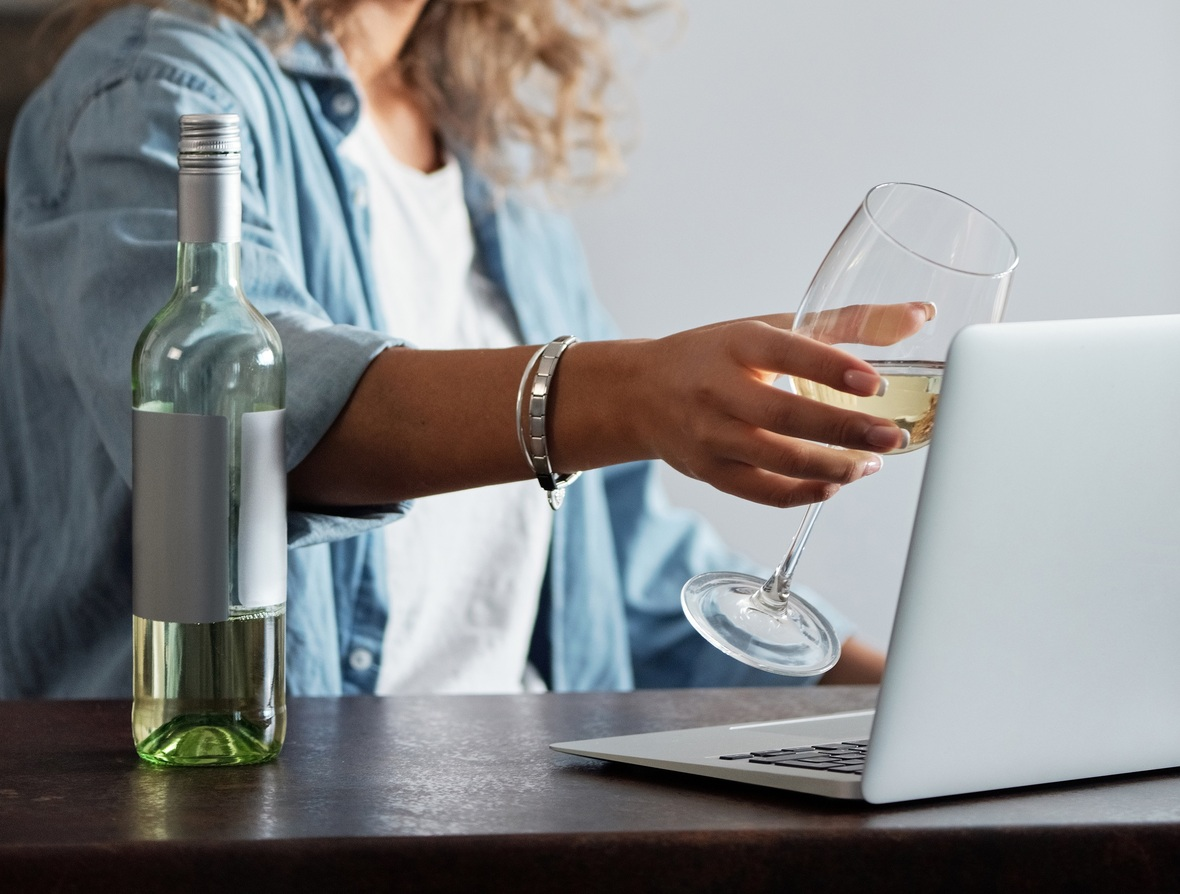If you were unable to join us for any of our past events, please consider supporting any of these fabulous brands and professionals by buying their services or products, subscribing to their Podcasts and following their social media. This community is one big family and we support one another!

These were the most recent guests Shall We Wine hosted and who we hosted them with:/* styles */ CLICK BELOW
 table div table+table+table+table+table+table+table+table+table+table+table+table+table+table+table+table+table+table+table+table+table+table+table+table+table+table+table+table+table+table div table{width:100%;padding:0}table div table+table+table+table+table+table+table+table+table+table+table+table+table+table+table+table+table+table+table+table+table+table+table+table+table+table+table+table+table+table div table table{padding:0;float:left!important;width:26.059%!important}table div table+table+table+table+table+table+table+table+table+table+table+table+table+table+table+table+table+table+table+table+table+table+table+table+table+table+table+table+table+table div table td{padding-left:29px;padding-right:29px}table div table+table+table+table+table+table+table+table+table+table+table+table+table+table+table+table+table+table+table+table+table+table+table+table+table+table+table+table+table+table div table table+table,table div table+table+table+table+table+table+table+table+table+table+table+table+table+table+table+table+table+table+table+table+table+table+table+table+table+table+table+table+table+table div table table+table+table{float:left!important;width:26.059%!important}table div table+table+table+table+table+table+table+table+table+table+table+table+table+table+table+table+table+table+table+table+table+table+table+table+table+table+table+table+table+table div table table td,table div table+table+table+table+table+table+table+table+table+table+table+table+table+table+table+table+table+table+table+table+table+table+table+table+table+table+table+table+table+table div table table+table td,table div table+table+table+table+table+table+table+table+table+table+table+table+table+table+table+table+table+table+table+table+table+table+table+table+table+table+table+table+table+table div table table+table+table td{padding-left:0;padding-right:20px}table div table+table+table+table+table+table+table+table+table+table+table+table+table+table+table+table+table+table+table+table+table+table+table+table+table+table+table+table+table+table div table table+table+table+table{float:left!important;width:21.822999999999993%!important}table div table+table+table+table+table+table+table+table+table+table+table+table+table+table+table+table+table+table+table+table+table+table+table+table+table+table+table+table+table+table div table table+table+table+table td{padding-left:0;padding-right:0}/* styles */
 table div table+table+table+table+table+table+table+table+table+table+table+table+table+table+table+table+table+table+table+table+table+table+table+table+table+table+table+table+table+table+table div table{width:100%;padding:0}table div table+table+table+table+table+table+table+table+table+table+table+table+table+table+table+table+table+table+table+table+table+table+table+table+table+table+table+table+table+table+table div table img{width:96.23%;padding:0;float:none}table div table+table+table+table+table+table+table+table+table+table+table+table+table+table+table+table+table+table+table+table+table+table+table+table+table+table+table+table+table+table+table div table td{width:100%;padding:0 1.88% 18px}/* styles */## WINE OF THE WEEK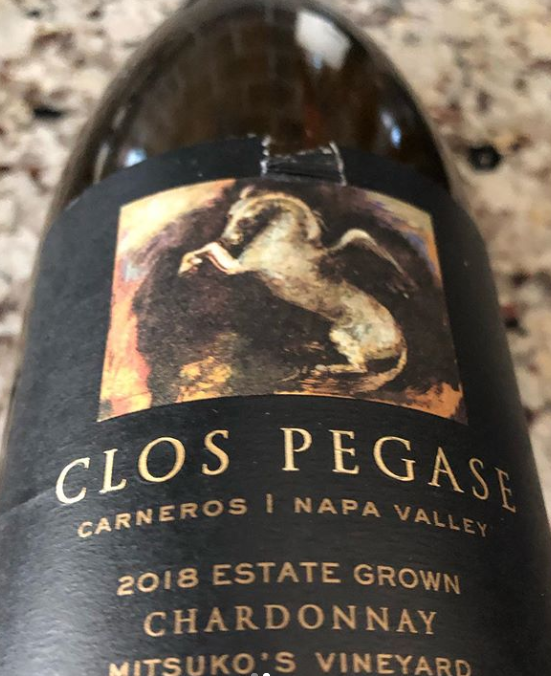We are ABC: All About Chardonnay! Pour yourself a glass of sunshine. The 2018 Clos Pegas Estate Grown Chardonnay has a bright happy-yellow color. Your mood is instantly lifted. The palate made me as giddy.

A polished palate with flavors of melon, grilled peach and toast. Simply delicious!

 table div table+table+table+table+table+table+table+table+table+table+table+table+table+table+table+table+table+table+table+table+table+table+table+table+table+table+table+table+table+table+table+table+table+table div table{width:100%;padding:0}table div table+table+table+table+table+table+table+table+table+table+table+table+table+table+table+table+table+table+table+table+table+table+table+table+table+table+table+table+table+table+table+table+table+table div table img{width:96.23%;padding:0;float:none}table div table+table+table+table+table+table+table+table+table+table+table+table+table+table+table+table+table+table+table+table+table+table+table+table+table+table+table+table+table+table+table+table+table+table div table td{width:100%;padding:0 1.88% 18px}/* styles */## TRY THIS AT HOME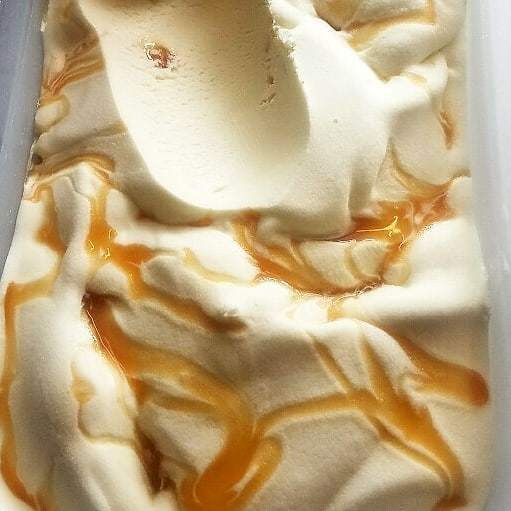During our cooking demo last week with Chef Brad Anderson, we featured a special dessert. It's so good that it needs to be repeated! For our demo we had paired specialty cocktails but we have a special wine pairing below for this newsletter.

YUM!

 /* styles */ SPICED BANANA ICE CREAM WITH QUICK CARAMEL SAUCE 4-6 frozen bananas cut into med pieces 1/4 tsp cinnamon 1/4 tsp nutmeg 1/4 tsp dried ginger Optional: 1/8 C choice type of milk(whole milk, heavy cream,1/2&1/2, almond or coconut milk) Sauce: 1 C brown sugar (light or dark) 1/2 C butter 1/4 C milk 1 tsp vanilla extract Pinch of salt Equipment Needed: Electric blender Small sauce pan Rubber spatula Wooden spoon Directions: In a blender add bananas and dried spices. Blend until smooth creamy. Add milk to mixture if it’s not coming together. Using a rubber spatula put ice cream mixture into a bowl. Cover and place in freezer. For the Sauce: Put all ingredients into a small sauce pan and place over medium heat. Using a whisk stir ingredients until well Combined. Watch the mixture until it starts to slowly boil. Turn off heat and stir until smooth. Using a rubber spatula pour into a small bowl and set aside to cool. When ready for dessert place desired amount of ice cream in a bowl and top with caramel sauce. Add some toasted almond, toasted coconut, and /or chocolate chips for added crunch and flavor!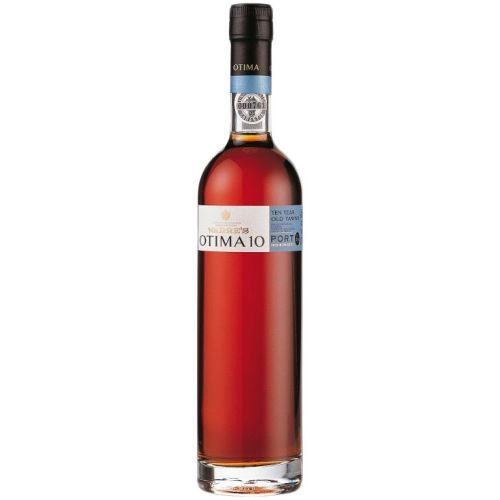Wine Pairing:
Warres Otima 10 Year Tawny Port

This is delicate, Non-Vintage Port in a lighter, Tawny style from one of Portugal's distinguished Port houses. A good go-to if you're not trying to get into the heavy depth of a Vintage Port or the fruit-forwardness of Ruby Port at the moment.

It is extremely versatile and works even during these summer weather months, and offers comforting nutty and toffee qualities.

 table div table+table+table+table+table+table+table+table+table+table+table+table+table+table+table+table+table+table+table+table+table+table+table+table+table+table+table+table+table+table+table+table+table+table+table+table+table+table+table div table{width:100%;padding:0}table div table+table+table+table+table+table+table+table+table+table+table+table+table+table+table+table+table+table+table+table+table+table+table+table+table+table+table+table+table+table+table+table+table+table+table+table+table+table+table div table img{width:96.23%;padding:0;float:none}table div table+table+table+table+table+table+table+table+table+table+table+table+table+table+table+table+table+table+table+table+table+table+table+table+table+table+table+table+table+table+table+table+table+table+table+table+table+table+table div table td{width:100%;padding:0 1.88% 18px}/* styles */## BOOK A PRIVATE OR CORPORATE COCKTAIL HOUR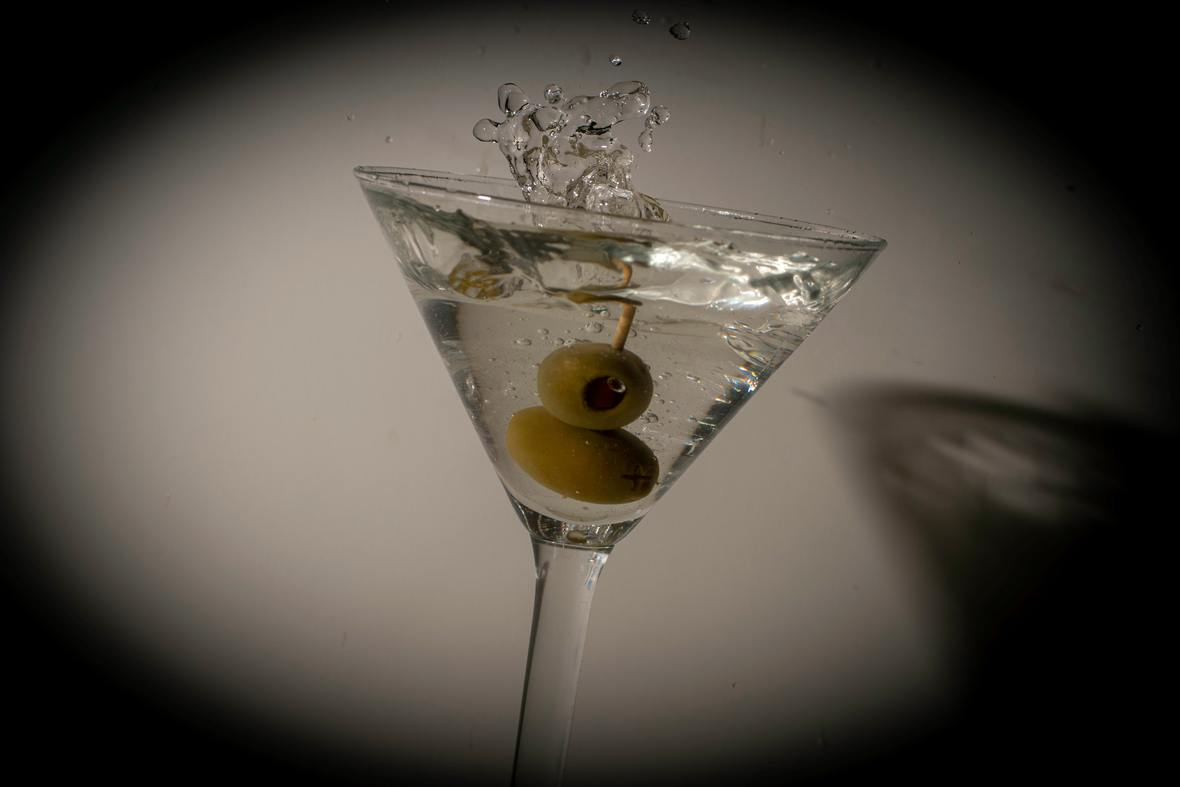Maybe you want to host a cocktail hour for a small group of friends.

Or maybe you want to throw a cocktail hour to celebrate a birthday or special milestone.

Or maybe you're tired of hosting the same kind of virtual events for your team and are looking for something to make them different and engage them.

Whatever the case is, you've come to the right place. Just as Shall We Wine does in-person experiences, we also plan experiences for corporate virtual events. We work with our corporate clients to create wine and spirit-focused experiences!

 table div table+table+table+table+table+table+table+table+table+table+table+table+table+table+table+table+table+table+table+table+table+table+table+table+table+table+table+table+table+table+table+table+table+table+table+table+table+table+table+table+table+table+table div table{width:100%;padding:0}table div table+table+table+table+table+table+table+table+table+table+table+table+table+table+table+table+table+table+table+table+table+table+table+table+table+table+table+table+table+table+table+table+table+table+table+table+table+table+table+table+table+table+table div table img{width:96.23%;padding:0;float:none}table div table+table+table+table+table+table+table+table+table+table+table+table+table+table+table+table+table+table+table+table+table+table+table+table+table+table+table+table+table+table+table+table+table+table+table+table+table+table+table+table+table+table+table div table td{width:100%;padding:0 1.88% 18px}/* styles */## DID YOU KNOW...Top 100 Wine Blogs, Websites & Influencers in 2020

Feedspot featured Shall We Wine as one of the Top 100 Wine Blogs, Websites & Influencers in 2020! Thank you from the bottom of our hearts, Shall We Wine supporters.

Here you will find things like our Wine of the Week and other special wine & spirits topics! Stay in the know and read up on some of the cool things we've been sharing.

 table div table+table+table+table+table+table+table+table+table+table+table+table+table+table+table+table+table+table+table+table+table+table+table+table+table+table+table+table+table+table+table+table+table+table+table+table+table+table+table+table+table+table+table+table+table+table div table{width:100%;padding:0}table div table+table+table+table+table+table+table+table+table+table+table+table+table+table+table+table+table+table+table+table+table+table+table+table+table+table+table+table+table+table+table+table+table+table+table+table+table+table+table+table+table+table+table+table+table+table div table img{width:96.23%;padding:0;float:none}table div table+table+table+table+table+table+table+table+table+table+table+table+table+table+table+table+table+table+table+table+table+table+table+table+table+table+table+table+table+table+table+table+table+table+table+table+table+table+table+table+table+table+table+table+table+table div table td{width:100%;padding:0 1.88% 18px}/* styles */## ** SUPPORT SHALL WE WINE DURING QUARANTINE **

 /* styles */
 /* styles */ For yourself or as a gift... it's one way you can support us!/* styles */ As both a sommelier and poet, I see wine elevated by words and vice versa. On the surface, wine and poetry can seem unrelated. Searching for Cloves & Lilies: The Wine Edition is one way of showing the congruence of the two. Seemingly different mediums can be more intertwined than you think. Challenge yourself to see likeness, positivity and common ground. A collection of original poems from Regine T. Rousseau, the book Searching for Cloves and Lilies: The Wine Edition explores pairing wine with poetry. The idea of pairing both wine and poetry was initially met with confused looks. But a national book tour and some new friends later, the Shall We Wine tribe has expanded into a think-outside-the-box wine pairing bunch! How amazing. We love wine, pass it on!
 table div table+table+table+table+table+table+table+table+table+table+table+table+table+table+table+table+table+table+table+table+table+table+table+table+table+table+table+table+table+table+table+table+table+table+table+table+table+table+table+table+table+table+table+table+table+table+table+table+table+table+table+table+table div table{width:100%;padding:0}table div table+table+table+table+table+table+table+table+table+table+table+table+table+table+table+table+table+table+table+table+table+table+table+table+table+table+table+table+table+table+table+table+table+table+table+table+table+table+table+table+table+table+table+table+table+table+table+table+table+table+table+table+table div table img{width:96.23%;padding:0;float:none}table div table+table+table+table+table+table+table+table+table+table+table+table+table+table+table+table+table+table+table+table+table+table+table+table+table+table+table+table+table+table+table+table+table+table+table+table+table+table+table+table+table+table+table+table+table+table+table+table+table+table+table+table+table div table td{width:100%;padding:0 1.88% 18px}/* styles */## THANKS AND TOGETHERNESSIn an effort to maintain social distancing measures, Shall We Wine will continue to devote our time to connecting with each and every one of our friends and supporters like you virtually. We're constantly sharing fun content to help brighten up your weeks!

We appreciate your patience and cooperation as we work through these times together. Thank you for your continued support in making Shall We Wine your go-to wine & lifestyle resource!/* styles */ Learn, Drink, Repeat! The Shall We Wine Team
 Like   Tweet   Pin   +1   in